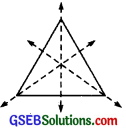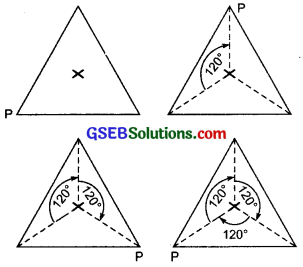# GSEB Solutions Class 7 Maths Chapter 14 Symmetry Ex 14.3

Gujarat Board GSEB Textbook Solutions Class 7 Maths Chapter 14 Symmetry Ex 14.3 Textbook Questions and Answers.

## Gujarat Board Textbook Solutions Class 7 Maths Chapter 14 Symmetry Ex 14.3Question 1.
Name any two figures that have both line symmetry and rotational symmetry.
Solution:An equilateral triangle and circle have both line symmetry and rotational symmetry.

Question 2.
Draw, wherever possible, a rough sketch of
(i) a triangle with both line and rotational symmetries of order more than 1.
(ii) a triangle with only line symmetry and no rotational symmetry of order more than 1.
(iii) a quadrilateral with a rotational symmetry of order more than 1 but not a line symmetry,
(iv) a quadrilateral with line symmetry but not a rotational symmetry of order more than 1.
Solution:
(i) An equilateral triangle has 3 lines of symmetry. An equilateral triangle has rotational symmetry also of order 3.(ii) It is not possible to have such a triangle.(iii)Trapezium

(iv) Not possible.Question 3.
If a figure has two or more lines of symmetry, should it have rotational symmetry of order more than 1?
Solution:
Yes.

Question 4.
Fill in the blanks:Solution:Question 5.
Name the quadrilaterals which have both line and rotational symmetry of order more than 1.
Solution:
The square, rectangle and a rhombus are the quadrilaterals having both line symmetry and rotational symmetry.Question 6.
After rotating by 60° about a centre, a figure looks exactly the same as its original position. At what other angles will this happen for the figure?
Solution:
The figure will look exactly the same as its original position at: 120°, 180°, 240°, 300°, and 360°.

Question 7.
Can we have a rotational symmetry of order more than 1 whose angle of rotation is
(i) 45°?
(ii) 17°?
Solution:
(i) Yes
(ii) No.